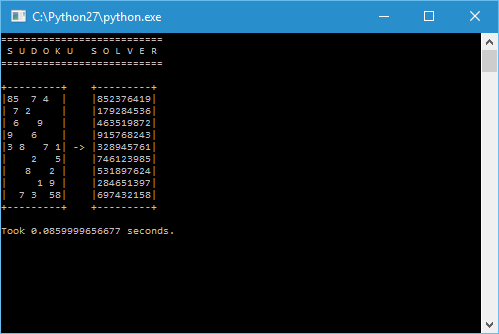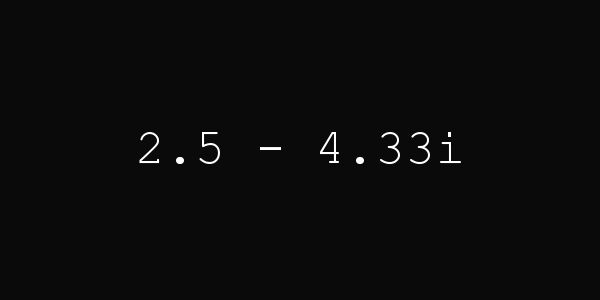# Web Sudoku Solver

2016-08-13, post № 137

Auto Hotkey, games, programming, Python, #AHK, #automatic, #fast times, #good times, #web, #Web Sudoku

In my last post I used Web Sudoku to get a Sudoku as an example for my solver.

After that I wanted to automate the process of looking up a Sudoku, solving it and typing it in. But while trying to get the Sudoku’s numbers, I noticed that the whole, solved Sudoku was stored in plaintext! (Look at this page’s source code.)
So I just needed to get that information, open the Web Sudoku page in a browser and type in the already solved Sudoku.

To accomplish said goal I used the python module urllib to get the Web Sudoku page’s source code and the module webbrowser to open the page in a browser. To type in the Sudoku I used AutoHotkey.

The finished program takes a level (easy, medium, hard or evil) and an id (the Sudoku’s identification number) to get a Sudoku, create an AHK file, execute it and open a web browser.
All you have to do is to click into the first box, press a key (‘F1’ in this case) and the Sudoku gets solved! You then just need to wait a minute, which is the minimum time Web Sudoku wants you to take to solve a Sudoku, and the AHK script hits enter.

You can get really good times with this:

Source code: web-sudoku-solver.py

# Sudoku Solver

2016-08-06, post № 136

programming, Python, #666, #evil, #logic, #solve, #solving, #Web Sudoku

This program solves a given Sudoku.
It uses a simple strategy, looking at each box and determining those numbers that are not in its row, column and square. If that list has length 𝟣, the box’s number is determined.
After going through each box, the program generates child Sudokus in which the first empty box get filled with one of the possible numbers for that box. Sudokus with an error get eliminated.
Using this simple but effective strategy this program can solve hard Sudokus in under a second. As an example, I used Evil Puzzle 666 from Web Sudoku.Source code: sudoku-solver.py

# JClock VIII

2016-07-30, post № 135

programming, Pygame, Python, #clock, #complex number, #hour, #minute, #plane, #time

Interpreting the hour hand on a clock as a two-dimensional object on a plane, the hand’s tip can be seen as a complex number.
This clock converts the hour hand’s position into a complex number, sets the number’s length to the current minutes and displays it in the form$a+b\cdot i$.
The angle 𝜑 is determined by the hours passed ($\frac{2\cdot\pi\cdot\text{hour}}{12}=\frac{\pi\cdot\text{hour}}{6}$) but has to be slightly modified because a complex number starts at the horizontal axis and turns anti-clockwise whilst an hour hand starts at the vertical axis and turns — as the name implies — clockwise. Thus,$\varphi=(2\cdot\pi-\frac{\pi\cdot\text{hour}}{6})+\frac{\pi}{2}=(\frac{15-\text{hour}}{6})\cdot\pi$.

The complex number’s length is simply determined by the minutes passed. Because the length must not be equal to 𝟢, I simply add 𝟣:$|z|=k=\text{minute}+1$.
Lastly, to convert a complex number of the form$k\cdot e^{\varphi\cdot i}$ into the form$a+b\cdot i$, I use the formula$k\cdot(\cos{\varphi}+\sin{\varphi}\cdot i)=a+b\cdot i$.Source code: jclock-viii.py

# Weekday

2016-07-23, post № 134

programming, Python, #calculation, #date, #day, #month, #time, #year

Determining the weekday based on a date composed of day, month and year.
The program counts up all the days from the 1st of January 1 to the given date, divides it by 𝟩, looks at the remainder and returns the weekday.

Source code: weekday.py

# Triangular Squares

2016-07-16, post № 133

programming, Python, Wolfram Language, #equation, #number, #number theory, #numbers, #OEIS, #square, #triangle, #triangles

In a recent video, Matt Parker showed a triangular number that also is a square number, 𝟨, and asked if there were more.

A triangular number has the form$\frac{n^2+n}{2}$ — shown by Euler — and a square number has the form$m^2$.
Triangular squares are those numbers for which$\frac{n^2+n}{2}=m^2$ with$n,m\in\mathbb{N}$.
Examples are$\{0,1,6,35,204,1189,6930,\dots\}$ (sequence A001109 in OEIS).

To check if triangular numbers are square numbers is easy (code listed below), but a mathematical function would be nicer.
The first thing I tried was to define the triangular number’s square root as a whole number,$\sqrt{\frac{n^2+n}{2}}=\lfloor\sqrt{\frac{n^2+n}{2}}\rfloor$. This function does not return the square numbers that are triangular but the triangular numbers that are square.
The resulting sequence is$\{0,1,8,49,288,1681,9800,\dots\}$ (sequence A001108 in OEIS).

Source code: triangular-squares.py

# RGB Jallenge

2016-07-09, post № 132

games, programming, Pygame, Python, #blue, #color, #colors, #colour, #colours, #green, #guess, #guessing, #red

This is a clone of The Great RGB Guessing Challenge . The challenge works like this: You are presented three numbers ranging from 𝟢 to 𝟤𝟧𝟧 representing a rgb color and three color bubbles. To get a point you must choose the color bubble corresponding to the rgb values. The more points you get, the higher your score.

## Controls

• Click on the bubble to choose it.
Source code: rgb-jallenge.py

# Palindrome Function

2016-07-02, post № 131

mathematics, programming, Python, #p(n), #sum

To get a number’s palindrome in a programming language like python is easy. There are ways to swap between integer and string and strings can be manipulated.

>>> n = 1234
>>> int(str(n)[::-1])
4321

But I wanted to create a mathematical function 𝑝(𝑛), which returns an integer’s palindrome. Thus 𝑝(𝟣𝟤𝟥𝟦) = 𝟦𝟥𝟤𝟣.

Firstly, I needed a way of determining the number’s size. In base 𝟣𝟢 the length is calculated using the logarithm to said base.Secondly, I need a way to isolate a specific digit. Using the floor function, this function returns the 𝑖-th digit (starting on the right with 𝑖 = 𝟢).Thirdly, both of these functions can be used to split up the number into a sum.Fourthly, I only need to swap the power of ten at the end to get my palindrome function.Thus the final function 𝑝(𝑛) is defined.To check if the formula is correct, I use 𝟣𝟤𝟥𝟦 (as seen above).# Jimon

2016-06-25, post № 130

games, programming, Pygame, Python, #color, #color memory, #memeory, #memory game, #remember, #sequence, #Simon, #Simon Says

This game is a recreation of the famous game Simon. In the game there are four colors which form a sequence that is expanding every cycle. The aim of the game is to memorize said sequence as far as possible.For more information on the Simon game visit this Wikipedia entry.

## Controls

• Click on the colored buttons to press them.
Source code: jimon.py
Jonathan Frech's blog; built 2021/10/02 17:36:09 CEST